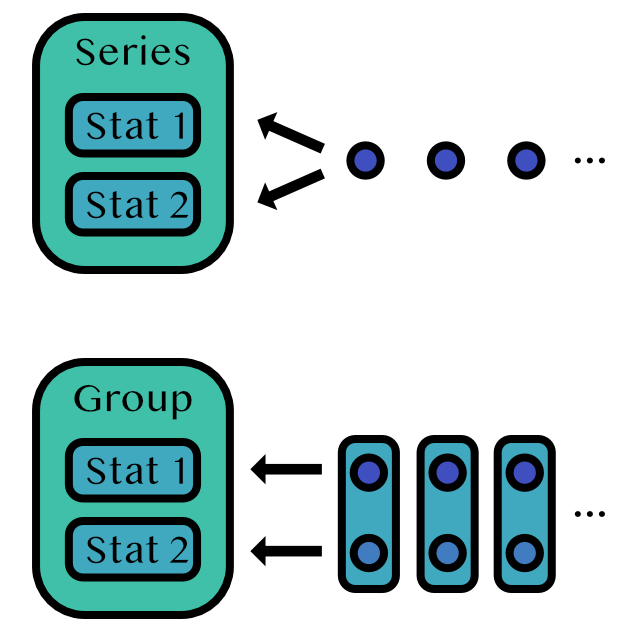# Collections of Stats

There are a few special OnlineStats that group together other OnlineStats: Series and Group.## Series

A Series tracks stats that should be applied to the same data stream.

y = rand(1000)

s = Series(Mean(), Variance())

fit!(s, y)
Series
├─ Mean: n=1_000 | value=0.505638
└─ Variance: n=1_000 | value=0.0864559


## Group

A Group tracks stats that should be applied to different data streams.

g = Group(Mean(), CountMap(Bool))

itr = zip(randn(100), rand(Bool, 100))

fit!(g, itr)
Group
├─ Mean: n=100 | value=-0.0541983
└─ CountMap: n=100 | value=OrderedDict{Bool, Int64}(0=>39, 1=>61)